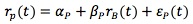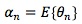### Why should I choose AnalystNotes?

AnalystNotes specializes in helping candidates pass. Period.

### Subject 1. The definition of alpha

Looking backward (ex post, or after the fact), alpha is the average of the realized residual returns.where,
rp(t): portfolio excess return.
rB(t): benchmark excess return.

The estimates of βP and αPobtained from the regression are the realized or historical β and α.

Looking forward (ex ante, or before the event), alpha is a forecast of residual return. This is the focus of the reading.where θn is residual return on stock n.

Alpha has the portfolio property. For example, the alpha of the portfolio which has two stocks can be estimated as follows:where hP(1) and hP(2) are holdings for stock 1 and 2, respectively.

By definition, the benchmark portfolio, risk-free portfolio and cash portfolio all have a residual return of zero. That is, alphas are benchmark-neutral.

#### Practice Question 1

Which portfolio has a zero residual return?

I. benchmark portfolio.
II. risk-free portfolio.
III. cash portfolio.

Correct Answer: I, II and III

#### Practice Question 2

Alpha, on ex ante basis, reflects:

A. average of excess returns.
B. forecast of residual return.
C. historical expected return.

#### Practice Question 3

If we have a two-stock holding with holdings hP(1) in stock 1 (with α1) and hP(2) in stock 2 (with α2), the alpha of the portfolio will be:

A. hP(1) α1 + hP(2) α2.
B. α1 x α2.
C. cannot be calculated based on the data given.